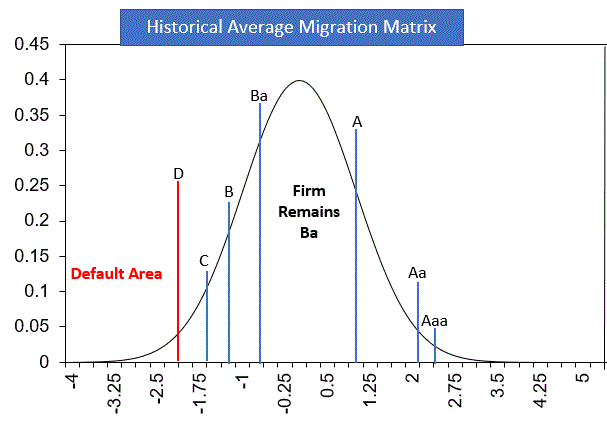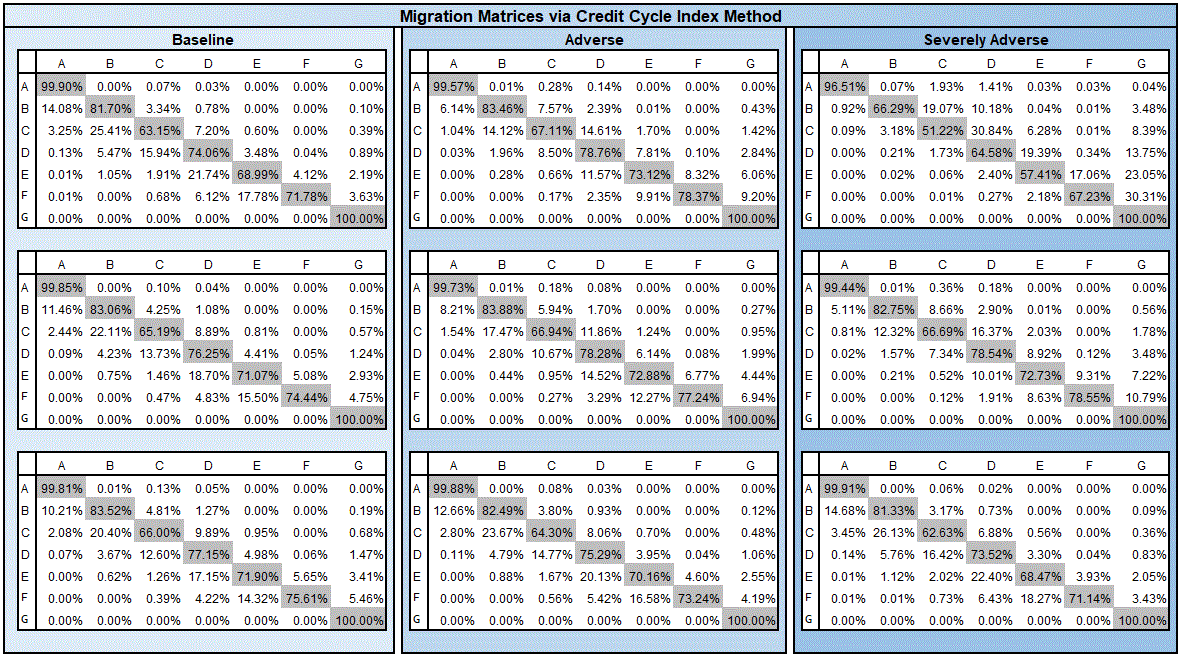Machine Learning and Credit Risk (part 2) – Credit Cycle Method

The canonical method to forecasting a credit migration matrix is an econometric model: the one factor approach described in Belkin et al. (1998). This approach suggests that one might consider an approach to condition migration (transition) matrices by creating a systematic component which represents the “credit cycle” that relates the economic condition to the credit quality of a loan portfolio.  The credit cycle can be thought of as the historical pattern of credit rating shared by all borrowers in a sector or economy.

The Vasicek (1987) Single Factor model presents a framework which, Forest, Belkin and Suchower (1998) used to develop the One-Parameter Representation method. In that model, migration behaviors are described by standard normal variables instead of transition probabilities without the loss of information. The transition through probabilities are transformed to thresholds where the upper and lower bounds of the threshold values together represent bins. Therefore, when a random variable falls within a bin that signifies a transition to the corresponding transition rating bucket.

The advantage of representing transitions probabilities in terms of the threshold framework is that we can use the standard normal density curve to understand the behavior rating transitions.

The area under a standard normal curve between the lower and upper bounds of a thresholds for a bin is the transition probability. Therefore, in the context of economic conditions, the shifting of curves (to the left or the right) under static thresholds, informs us about the behavior of transitions matrices during benign and stressed periods.

Figure 2. Graphical illustration of thresholds for representing transitions through a standard normal variable.To the extent that we can represent economic conditions with a single variable, the credit index, which allows us to ’shift’ the average or (Through-the-Cycle) transition matrix by this amount to generate a forecast of the transition matrix. The credit Index is thus a standardized index of credit quality change. Therefore, large positive (negative) credit index implies more upgrades (downgrades) in the portfolio.

Figure 3. Graphical illustration of shifting the distribution function to change transition probabilities.See Forest, Belkin and Suchower (1998) for a more detailed discussion.

Figure 4 displays the conditional matrices derived by the Credit Cycle Index approach. It displays the forecasted migration matrices conditioned on Baseline, Adverse, and Severely Adverse economic conditions.